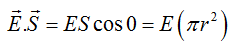# Concepts of Physics Ch-30 Gauss Law (SA)

1. A small plane area is rotated in an electric field. In which orientation of the area is the flux of electric field through the area maximum? In which orientation is it zero?

Solution: The flux of an electric field E through a surface area dS is given by, dφ = E dS , where dφ is the flux. Therefore, dφ = E dS cosθ. Here, θ is the angle between the electric field E and the normal to the surface area.

Thus, for the flux to be the maximum cosθ should be maximum. Thus, for θ=0, the flux is maximum, i.e., the electric field lines are perpendicular to the surface area.

The flux is minimum if θ=90. Thus, cosθ=0 and, hence, flux is also 0. Thus, if the electric field lines are parallel to the surface area, the flux is minimum.

1. A circular ring of radius r made of a non-conducting material is placed with its axis parallel to a uniform electric field. The ring is rotated about a diameter through 180°. Does the flux of electric field change? If yes, does it decrease or increase?

Solution: It is given that the circular ring, made of a non- conducting material, of radius r is placed with its axis parallel to a uniform electric

field. This means that both the electric field and the area vector are parallel to each other (area vector is always perpendicular to the surface area). Thus, the flux through the ring is given by,Now, when the ring is rotated about its diameter through 180, flux becomes -E(πr2)

1. A charge Q is uniformly distributed on a thin spherical shell. What is the field at the centre of the shell? If a point charge is brought close to the shell, will the field at the centre change? Does your answer depend on whether the shell is conducting or non-conducting?

Solution: The field at the centre of the shell is zero. As all the charge given to a conductor resides on the surface, the field at any point inside the conducting sphere is zero. Also, the charge distribution at the surface is uniform, so all the electric field vectors due to these charges at the centre are equal and opposite. So, they cancel each other, resulting in a zero net value of the field.

When a charge is brought near the shell, due to induction, opposite polarity charges induce on the surface nearer to the charge and the same polarity charges appear on the face farther from the charge. In this way, a field is generated inside the shell. Hence, the field at the centre is non- zero.

Yes, our answer changes in case of a non- conducting spherical shell. As the charge given to the surface of a non- conducting spherical shell spreads non- uniformity, there is a net electric field at the centre of the sphere.

1. A spherical shell made of plastic, contains a charge Q distributed uniformly over its surface. What is the electric field inside the shell? If the shell is hammered to de-shape it without altering the charge, will the field inside be changed? What happens if the shell is made of a metal?

Solution: As the shell is made of plastic, it is non- conducting. But as the charge is distributed uniformly over the surface of the shell, the sum of all the electric field vectors at the centre due to this kind of distribution is zero. But when the plastic shell is deformed, the distribution of charge on it becomes non- uniform. In other words, the sum of all the electric field vectors is non- zero now or the electric field exists at the centre now. In case of a deformed conductor, the field inside is always zero.

1. A point charge q is placed in a cavity in a metal block. If a charge Q is brought outside the metal, will the charge q feel an electric force?

Solution: Yes, the charge Q will feel an electric force, as the charge q given to the metal block appears on the surface. Hence, it exerts an electric force on the charge Q.

1. A rubber balloon is given a charge Q distributed uniformly over its surface. Is the field inside the balloon zero everywhere if the balloon does not have a spherical surface?

Solution: No, the field is not zero everywhere, as the electric field vector due to the charge distribution does not cancel out at any place inside the balloon because of its non spherical shape.

1. It is said that any charge given to a conductor comes to its surface. Should all the protons come to the surface? Should all the electrons come to the surface? Should all the free electrons come to the surface?

Solution: Protons never take part in any electrical phenomena because they are inside the nuclei and are not able to interact easily. These are the free electrons that are responsible for all electrical phenomena. So, if a conductor is given a negative charge, the free electrons come to the surface of the conductor. If the conductor is given a positive charge, electrons move away from the surface and leave a positive charge on the surface of the conductor.

Announcements

Join our Online JEE Test Series for 499/- Only (Web + App) for 1 Year

Join our Online NEET Test Series for 499/- Only for 1 Year